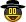## Recommended Posts

Hi everyone,

This is my special pet project.

It is an example of how u can use autoit to control external devices.

this script is made to comunicate with the MAX335 chip  using the SPI protocol via the LPT(printer) port

the beauty of the MAX335 chip is that the Clock, Data_In and the Chip_Select pins can be directly connected to the LPT port without any external components, and the 12V and 5V directly from an ATX PC power suply.

aditionaly i made a custom GUI with CommandFusion instaled on an Android Tablet that sends TCP commands to an Autoit TCP server that controls three dasy chained MAX335 chips that totals 24 independent NO switches

this script works perfectly for me

i just finished this project and i think i will make an UDF including more SPI devices

```\$DLLFileAndPath = @ScriptDir & "/inpout32.dll"

Global \$335_device_number=3 ;number of daisy chained chips
Global \$335_clock_bit=0 ;bit number for LPT pin 1 in the control Register of the LPT port(where i connected the CLK pin on te MAX335)
Global \$335_cs_bit=4;bit number for LPT pin 6 in the data Register of the LPT port(where i connected the CS pin on te MAX335)
Global \$335_data_in_bit=7;bit number for LPT pin 9 in the data Register of the LPT port(where i connected the DI pin on te MAX335)
Global \$clock_delay=1;this limits the clock speed but it works fine with 0

;the ini file will be created and will keep the MAX335 pins statuses
For \$i=0 To \$335_device_number*8-1 Step 1
IniWrite("355_buffer.ini","present_data",\$i,"0")
Next

set_max335_output(2,3,1) ; this will activate switch 2 on device 3 on the daisy chain

Func set_max335_output(\$output_no,\$device_no,\$status)
\$bit_no=(\$device_no-1)*8+\$output_no-1 ; get the exact bit number of the switch
IniWrite("355_buffer.ini","present_data",\$bit_no,\$status); whrite it to the buffer
;this part is where the SPI protocol begins
set_control_bit(\$335_clock_bit,1); drop CLK
set_data_bit(\$335_cs_bit,0) ;activate CS
Sleep(\$clock_delay)
For \$i=\$335_device_number*8-1 To 0 Step -1; start writing from buffer MostSignificantByte first
set_data_bit(\$335_data_in_bit,\$data) ; set data bit value
set_control_bit(\$335_clock_bit,0) ;raise CLK
Sleep(\$clock_delay)
set_control_bit(\$335_clock_bit,1); drop CLK
Sleep(\$clock_delay)
Next
set_data_bit(\$335_cs_bit,1);deactivate CS
EndFunc

Func set_data_bit(\$bit,\$stat=-1) ; it will write the value of the bit in the data reg of the LPT port
\$y= DllCall(\$DLLFileAndPath, "int", "Inp32", "int", "0x378")
\$bits=dec_to_bin(\$y)
If \$stat = -1 Then
If \$bits[\$bit]=0 Then
\$bits[\$bit]=1
Else
\$bits[\$bit]=0
EndIf
\$bcd=bin_to_dec(\$bits)
DllCall( \$DLLFileAndPath, "int", "Out32", "int", "0x378", "int", \$bcd)
Return 1
Else
If \$bits[\$bit]<>\$stat  Then
\$bits[\$bit]=\$stat
\$bcd=bin_to_dec(\$bits)
DllCall( \$DLLFileAndPath, "int", "Out32", "int", "0x378", "int", \$bcd)
Return 1
Else
Return 2
EndIf
EndIf
EndFunc

Func set_control_bit(\$bit,\$stat=-1); it will write the value of the bit in the control reg of the LPT port
\$y= DllCall(\$DLLFileAndPath, "int", "Inp32", "int", "0x37a")
\$bits=dec_to_bin(\$y)
If \$stat = -1 Then
If \$bits[\$bit]=0 Then
\$bits[\$bit]=1
Else
\$bits[\$bit]=0
EndIf
\$bcd=bin_to_dec(\$bits)
DllCall( \$DLLFileAndPath, "int", "Out32", "int", "0x37a", "int", \$bcd)
Return 1
Else
If \$bits[\$bit]<>\$stat  Then
\$bits[\$bit]=\$stat
\$bcd=bin_to_dec(\$bits)
DllCall( \$DLLFileAndPath, "int", "Out32", "int", "0x37a", "int", \$bcd)
Return 1
Else
Return 2
EndIf
EndIf
EndFunc

Func dec_to_bin(\$dec)
Local \$bit_array
If \$dec > 255 Then SetError(1,1,-1)
If \$dec < 0 Then SetError(2,1,-1)
For \$i=7 To 0 Step -1
If \$dec >= 2^\$i Then
\$bit_array[\$i] = 1
\$dec=\$dec-2^\$i
Else
\$bit_array[\$i] = 0
EndIf
Next
Return \$bit_array
EndFunc

Func bin_to_dec(\$bit_array)
If IsArray(\$bit_array) Then
If UBound(\$bit_array) = 8 Then
\$dec=0
For \$i=7 To 0 Step -1
\$dec = \$bit_array[\$i]*(2^\$i)+\$dec
Next
Else
SetError(2,1,-1)
EndIf
Else
SetError(1,1,-1)
EndIf
Return \$dec
EndFunc```
##### Share on other sites

• 2 weeks later...
• 5 months later...

thanks for this! looks like the most can be run in my program. using send bits is the important things in the real works.so we can build more auto machines!

but still hope autoit have its port api function groups may be can run it quickly.just like the LABVIEW.## Create an account

Register a new account

• ### Similar Content

• #### HttpApi UDF - HTTP Server API

By TheXman,

• 6,307 views
• #### AIDA64 - read shared memory data

By Irios,

• 0 replies
• 1,789 views
• #### Autoit-Socket-IO - Networking in AutoIt made simple! 1 2 3 4

By tarretarretarre,

• 60 replies
• 25,663 views
• #### Autoit-Socket-IO

By tarretarretarre,

• 3,540 views
• #### issues with ping to android phone

By mmoalem,

• 3 replies
• 1,070 views
×

• Wiki

• Back

• #### Beta

• Git
• FAQ
×
• Create New...